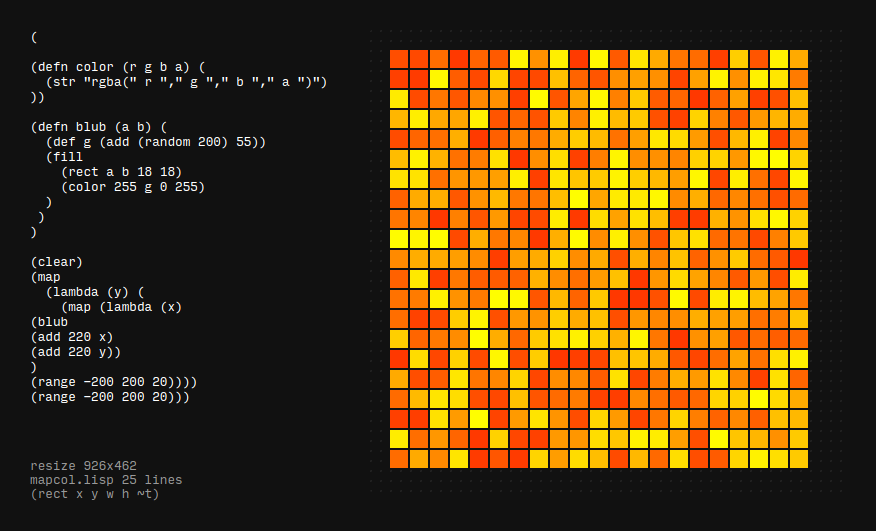## ronin experiment 2 - nested maps and colors

for this Ronin experiment I experimented with nested "map" commands and random fill colorsThis is the source code I used, but be aware that Ronin is under heavy development and things change quite fast currently

``````(

(defn color (r g b a) (
(str "rgba(" r "," g "," b "," a ")")
))

(defn blub (a b) (
(def g (add (random 200) 55))
(fill
(rect a b 18 18)
(color 255 g 0 255)
)
)
)

(clear)
(map
(lambda (y) (
(map (lambda (x)
(blub
(add (div (of (frame) "w") 2) x)
(add (div (of (frame) "h") 2) y))
)
(range -200 200 20))))
(range -200 200 20)))
``````